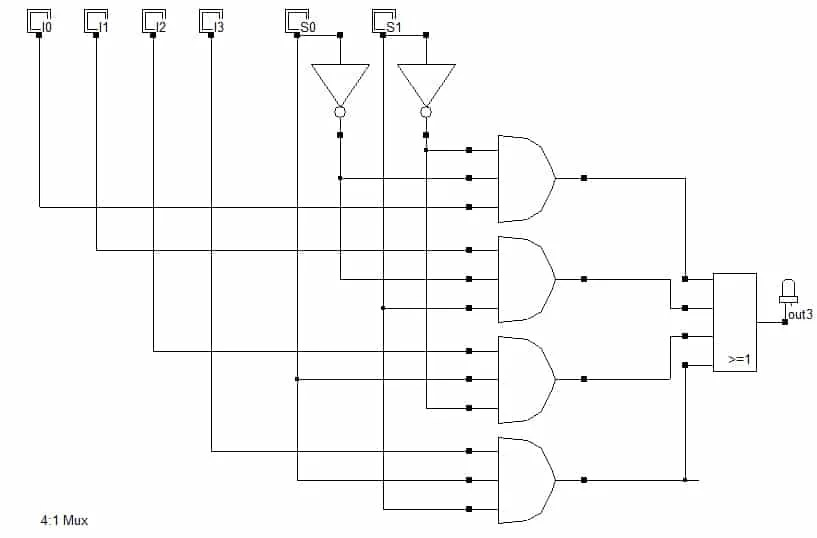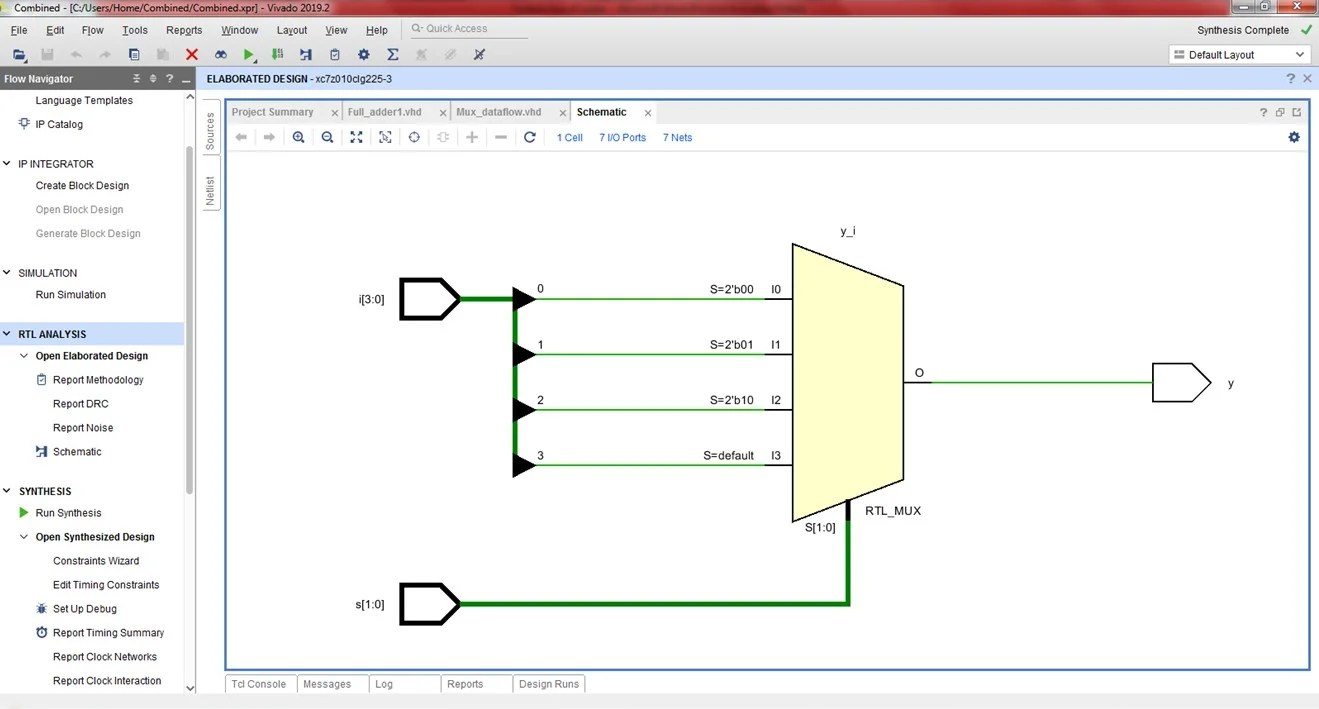View Course Path

# VHDL code for multiplexer using dataflow method – full code and explanation

In this post, we will take a look at implementing the VHDL code for a multiplexer using dataflow modeling. First, we will study the logic diagram and the truth table of the multiplexer and then the syntax of the VHDL code. After that, we will write a testbench to verify our code. We will also generate the RTL schematic and simulation waveforms.

Contents

## Explanation of the VHDL code for multiplexer using dataflow method. How does the code work?

A multiplexer is a combinational logic circuit that has several inputs, one output, and some select lines. At any instant, only one of the input lines is connected to the output. The input line is chosen by the value of the select inputs.

A general multiplexer is with n inputs, m select lines, and one output line is shown below.In this post, we will design a 4:1 multiplexer. From our post on multiplexers, we have the logic circuit and the truth table of a 4:1 multiplexer, as shown below.

### Logic circuit of a 4:1 Mux

A 4:1 mux has four inputs, two select lines, and one output.### Truth table of a 4:1 Mux

 I0 I1 I2 I3 S0 S1 Y I0 x x x 0 0 I0 x I1 x x 0 1 I1 x x I2 x 1 0 I2 x x x I3 1 1 I3

As we have seen in our post on dataflow architecture in VHDL, we have a special set of statements that allow us to work with circuits having select lines. These statements are exclusively useful when working with multiplexers and demultiplexers. We will use these when we write the VHDL code for demultiplexers too. With the with-select statements, you just need to assign the select signals to a vector of a suitable size. Here’s the syntax for that. First, the `entity` declaration:

```entity MUX4_1 is
Port ( i : in  STD_LOGIC_VECTOR (3 downto 0);
s : in  STD_LOGIC_VECTOR (1 downto 0);
y : out  STD_LOGIC);
end MUX4_1;

architecture dataflow of MUX4_1 is

begin
```

Then you have to assign the output port to the output values for every select input case. Here’s the syntax for that.

```with s select

y <=     i(0) when "00", --- commas at the end of each select output assignment and a semicolon to close off the last statement
i(1) when "01",
i(2) when "10",
i(3) when others;
```

## VHDL code for multiplexer using dataflow method

```library IEEE;
use IEEE.STD_LOGIC_1164.ALL;
use IEEE.STD_LOGIC_ARITH.ALL;
use IEEE.STD_LOGIC_UNSIGNED.ALL;

entity MUX4_1 is
Port ( i : in  STD_LOGIC_VECTOR (3 downto 0);
s : in  STD_LOGIC_VECTOR (1 downto 0);
y : out  STD_LOGIC);
end MUX4_1;

architecture dataflow of MUX4_1 is

begin

with s select
y <=     i(0) when "00",
i(1) when "01",
i(2) when "10",
i(3) when others;

end dataflow;```

## Testbench

To learn how to write a testbench in VHDL, refer to this guide.

```library IEEE;
use IEEE.STD_LOGIC_1164.ALL;
use IEEE.STD_LOGIC_ARITH.ALL;
use IEEE.STD_LOGIC_UNSIGNED.ALL;

entity Mux4_1_tb is
end entity;

architecture tb of Mux4_1_tb is
component MUX4_1 is
Port ( i : in STD_LOGIC_VECTOR (3 downto 0);
s : in STD_LOGIC_VECTOR (1 downto 0);
y : out STD_LOGIC);
end component;

signal i : STD_LOGIC_VECTOR (3 downto 0);
signal s : STD_LOGIC_VECTOR (1 downto 0);
signal y : STD_LOGIC;

begin

uut: MUX4_1 port map(
i => i,
s => s,
y => y);

stim: process
begin

i <= "1010";

s <= "00";
wait for 20 ns;

s <= "01";
wait for 20 ns;

s <= "10";
wait for 20 ns;

s <= "11";
wait for 20 ns;
wait;

end process;
end tb;```

## RTL Schematic## Simulation WaveformIf you have any comments or encounter any rogue errors, let us know in the comments section below.

Edit: Post updated with the testbench, RTL Schematic, and Simulation Waveform by Deepak Joshi.

This site uses Akismet to reduce spam. Learn how your comment data is processed.

Top